# Recursion Aaron Tan Recursion http www comp nus

• Slides: 84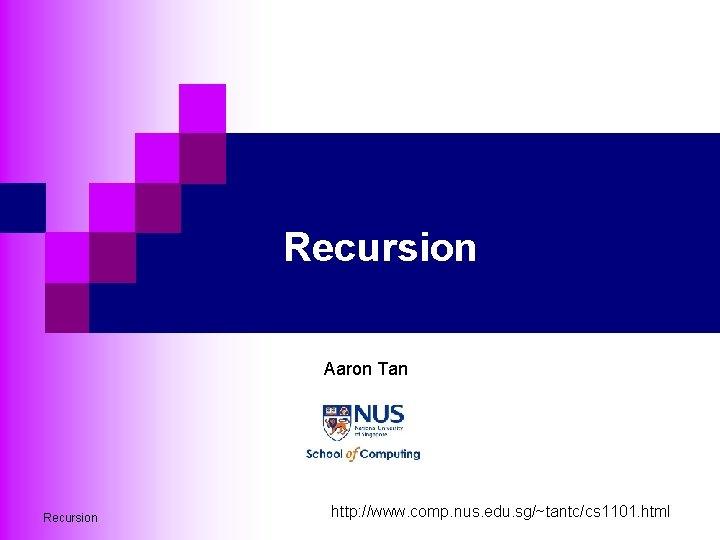Recursion Aaron Tan Recursion http: //www. comp. nus. edu. sg/~tantc/cs 1101. htmlRecursion + Recursion 2What is Recursion? (1/2) n To perform a task T by performing some similar smaller task(s) T’. n Example: ¨ Formula for factorial: n! = n * (n-1) * (n-2) * … * 2 * 1 for n > 0 0! = 1 ¨ Recursive formula for factorial: n! = n * (n– 1)! for n>0 0! = 1 (Examples: 3! = 6; 4! = 24; 7! = 5040) Recursion 3What is Recursion? (2/2) n The idea behind recursion is similar to PMI (Principle of Mathematical Induction). n Example: Prove the recursive formula for factorial. ¨ Basis: 0! = 1 ¨ Induction hypothesis: Assume that the recursive formula is true for x > 0. ¨ Inductive step: (x + 1)! = (x + 1) * x * (x – 1) * … * 1 = (x + 1) * x! Recursion 4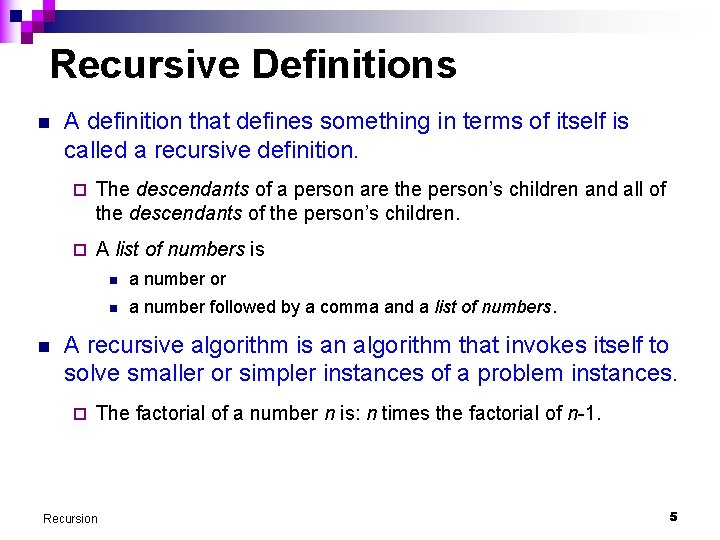Recursive Definitions n n A definition that defines something in terms of itself is called a recursive definition. ¨ The descendants of a person are the person’s children and all of the descendants of the person’s children. ¨ A list of numbers is n a number or n a number followed by a comma and a list of numbers. A recursive algorithm is an algorithm that invokes itself to solve smaller or simpler instances of a problem instances. ¨ The factorial of a number n is: n times the factorial of n-1. Recursion 5How to Multiply 6 by 3? (1/5) n Assume that we know only addition but not multiplication, except for the simplest case of x * 1 = x. n To employ recursion, we solve our problem by solving a smaller (but similar) problem, and then use the solution of this smaller problem to derive the solution of the original problem. Recursion 6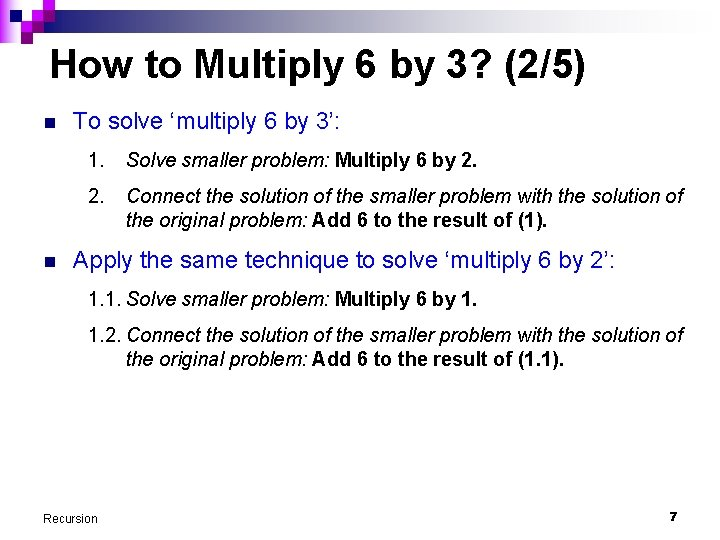How to Multiply 6 by 3? (2/5) n n To solve ‘multiply 6 by 3’: 1. Solve smaller problem: Multiply 6 by 2. Connect the solution of the smaller problem with the solution of the original problem: Add 6 to the result of (1). Apply the same technique to solve ‘multiply 6 by 2’: 1. 1. Solve smaller problem: Multiply 6 by 1. 1. 2. Connect the solution of the smaller problem with the solution of the original problem: Add 6 to the result of (1. 1). Recursion 7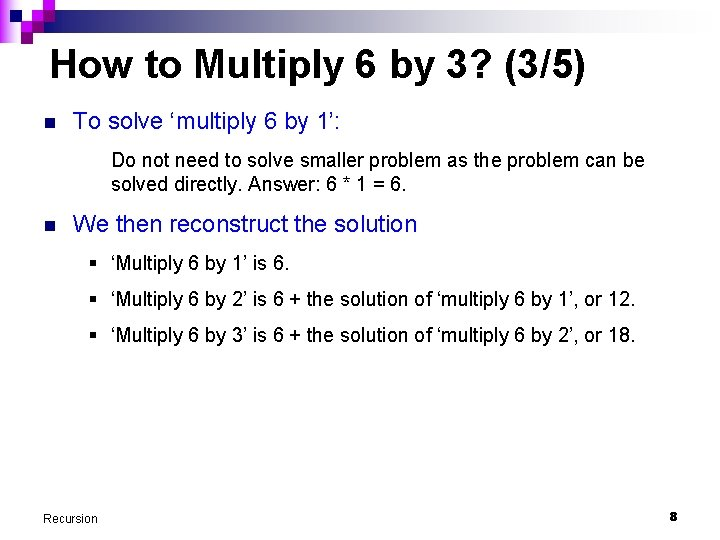How to Multiply 6 by 3? (3/5) n To solve ‘multiply 6 by 1’: Do not need to solve smaller problem as the problem can be solved directly. Answer: 6 * 1 = 6. n We then reconstruct the solution § ‘Multiply 6 by 1’ is 6. § ‘Multiply 6 by 2’ is 6 + the solution of ‘multiply 6 by 1’, or 12. § ‘Multiply 6 by 3’ is 6 + the solution of ‘multiply 6 by 2’, or 18. Recursion 8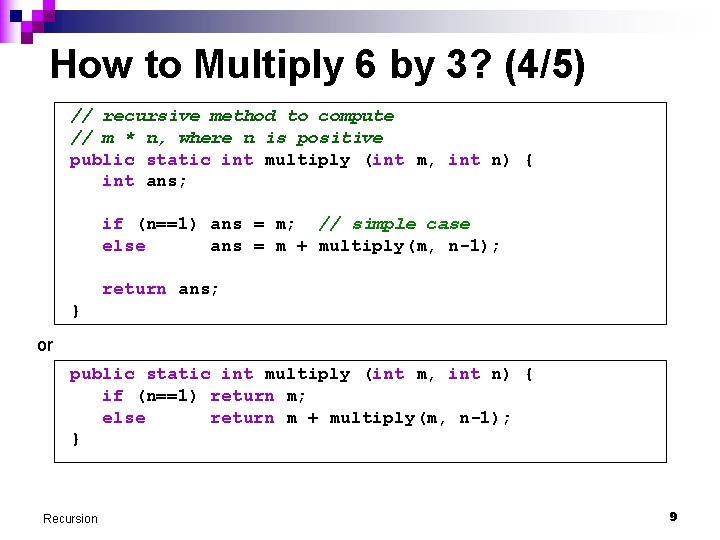How to Multiply 6 by 3? (4/5) // recursive method to compute // m * n, where n is positive public static int multiply (int m, int n) { int ans; if (n==1) ans = m; // simple case else ans = m + multiply(m, n-1); return ans; } or public static int multiply (int m, int n) { if (n==1) return m; else return m + multiply(m, n-1); } Recursion 9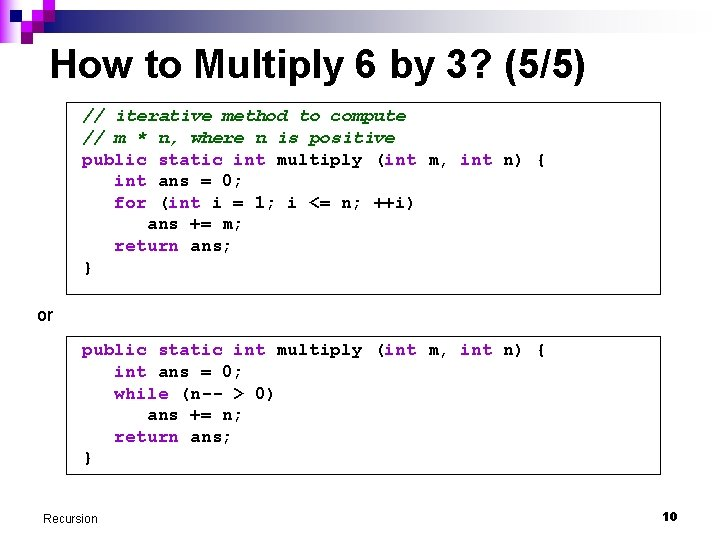How to Multiply 6 by 3? (5/5) // iterative method to compute // m * n, where n is positive public static int multiply (int m, int n) { int ans = 0; for (int i = 1; i <= n; ++i) ans += m; return ans; } or public static int multiply (int m, int n) { int ans = 0; while (n-- > 0) ans += n; return ans; } Recursion 10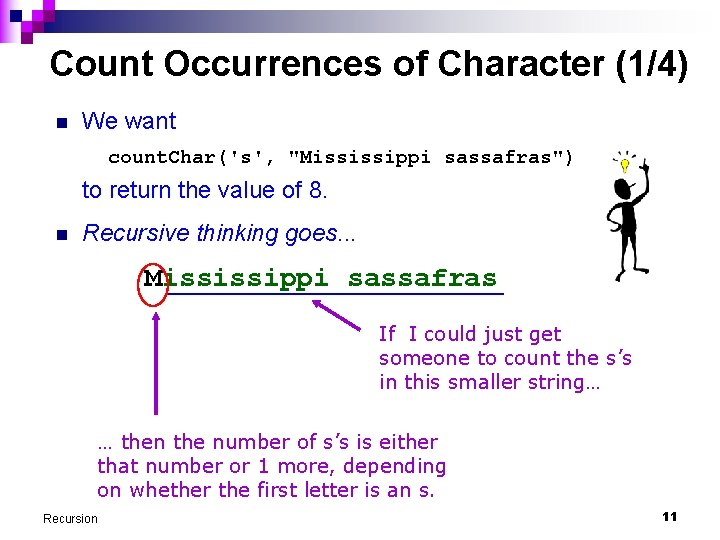Count Occurrences of Character (1/4) n We want count. Char('s', "Mississippi sassafras") to return the value of 8. n Recursive thinking goes. . . Mississippi sassafras If I could just get someone to count the s’s in this smaller string… … then the number of s’s is either that number or 1 more, depending on whether the first letter is an s. Recursion 11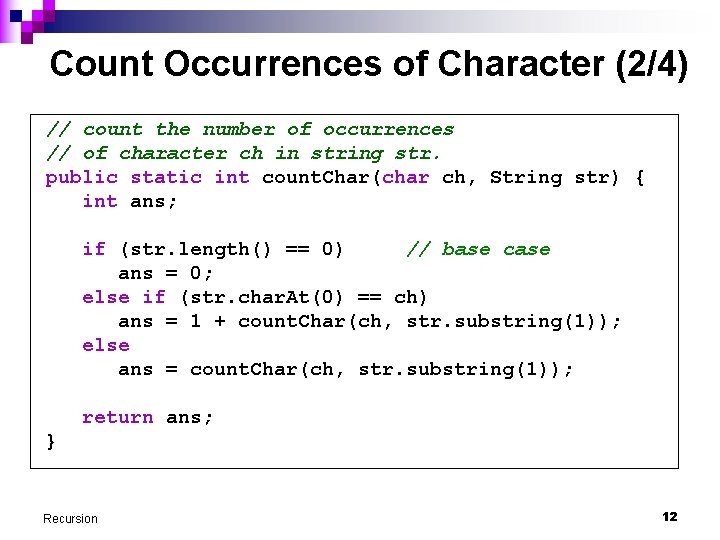Count Occurrences of Character (2/4) // count the number of occurrences // of character ch in string str. public static int count. Char(char ch, String str) { int ans; if (str. length() == 0) // base case ans = 0; else if (str. char. At(0) == ch) ans = 1 + count. Char(ch, str. substring(1)); else ans = count. Char(ch, str. substring(1)); return ans; } Recursion 12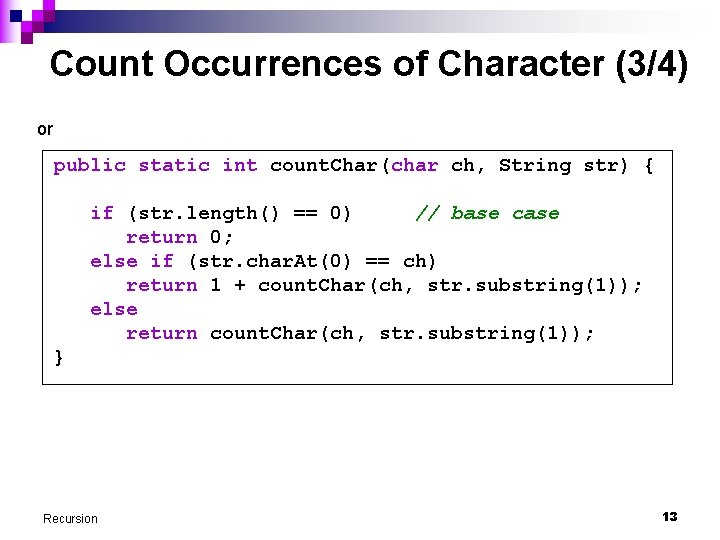Count Occurrences of Character (3/4) or public static int count. Char(char ch, String str) { if (str. length() == 0) // base case return 0; else if (str. char. At(0) == ch) return 1 + count. Char(ch, str. substring(1)); else return count. Char(ch, str. substring(1)); } Recursion 13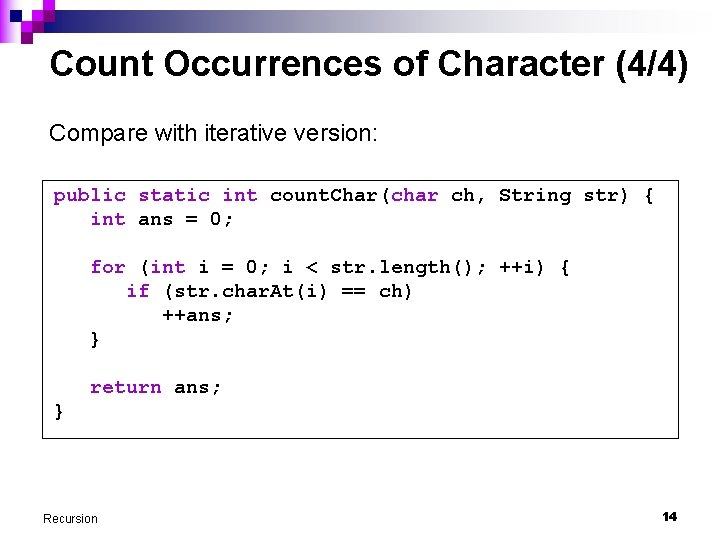Count Occurrences of Character (4/4) Compare with iterative version: public static int count. Char(char ch, String str) { int ans = 0; for (int i = 0; i < str. length(); ++i) { if (str. char. At(i) == ch) ++ans; } return ans; } Recursion 14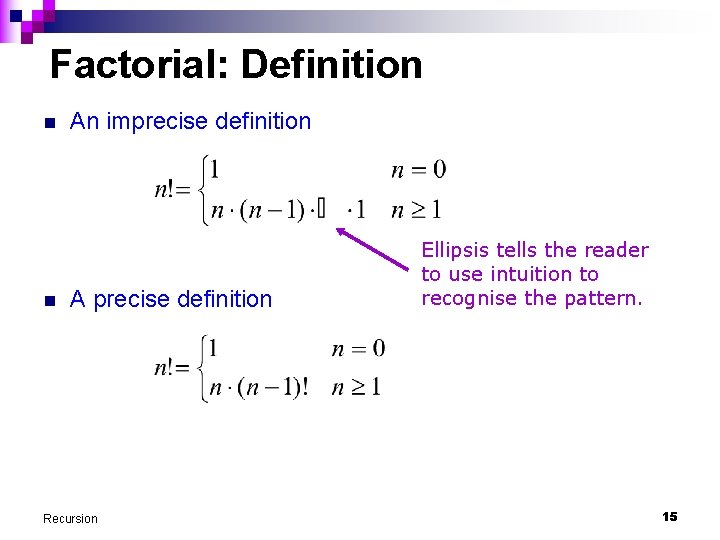Factorial: Definition n n An imprecise definition A precise definition Recursion Ellipsis tells the reader to use intuition to recognise the pattern. 15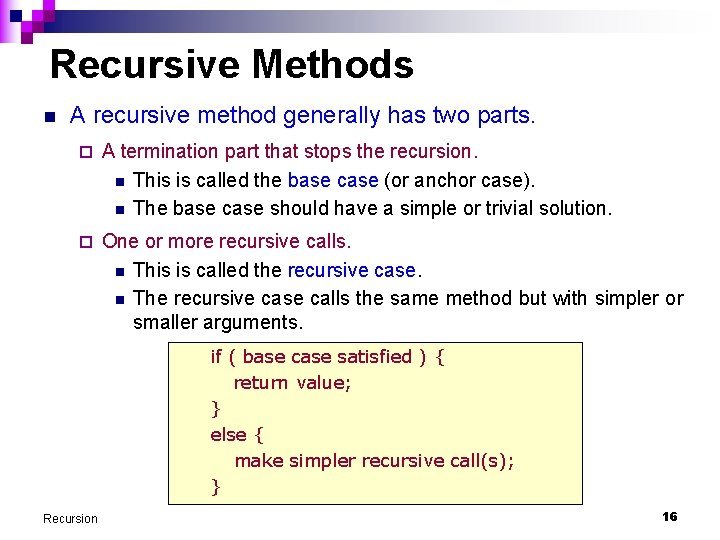Recursive Methods n A recursive method generally has two parts. ¨ A termination part that stops the recursion. n This is called the base case (or anchor case). n The base case should have a simple or trivial solution. ¨ One or more recursive calls. n This is called the recursive case. n The recursive case calls the same method but with simpler or smaller arguments. if ( base case satisfied ) { return value; } else { make simpler recursive call(s); } Recursion 16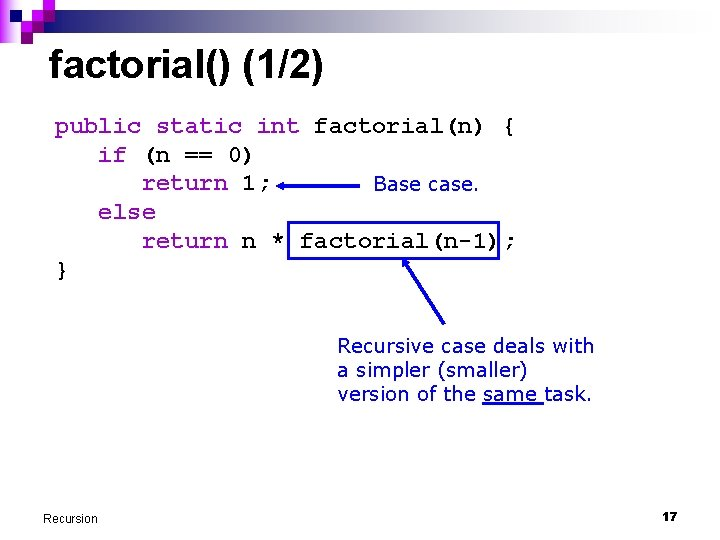factorial() (1/2) public static int factorial(n) { if (n == 0) return 1; Base case. else return n * factorial(n-1); } Recursive case deals with a simpler (smaller) version of the same task. Recursion 17factorial() (2/2) public static int factorial(n) { if (n == 0) return 1; else return n * factorial(n-1); } public static void main(String[] args) { Scanner scanner = new Scanner(System. in); int number = scanner. next. Int(); int nfactorial = factorial(number); System. out. println(number + "! = " + nfactorial); } Recursion 18Factorial: Recursive Invocation n A new activation record is created for every method invocation ¨ Including recursive invocations main() Recursion int nfactorial = factorial(n); factorial() n=3 return n * factorial(n-1); factorial() n=2 return n * factorial(n-1); factorial() n=1 return n * factorial(n-1); factorial() n=0 return 1; 19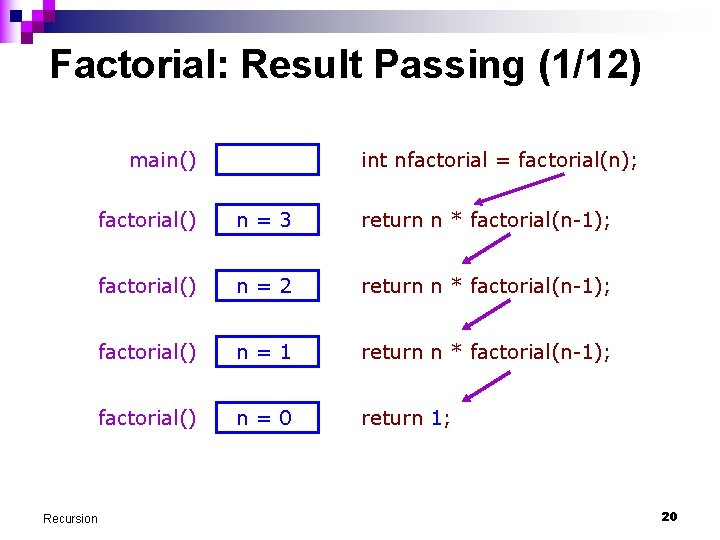Factorial: Result Passing (1/12) main() Recursion int nfactorial = factorial(n); factorial() n=3 return n * factorial(n-1); factorial() n=2 return n * factorial(n-1); factorial() n=1 return n * factorial(n-1); factorial() n=0 return 1; 20Factorial: Result Passing (2/12) main() Recursion int nfactorial = factorial(n); factorial() n=3 return n * factorial(n-1); factorial() n=2 return n * factorial(n-1); factorial() n=1 return n * factorial(n-1); factorial() n=0 return 1; 21Factorial: Result Passing (3/12) main() Recursion int nfactorial = factorial(n); factorial() n=3 return n * factorial(n-1); factorial() n=2 return n * factorial(n-1); factorial() n=1 return n * 1; 22Factorial: Result Passing (4/12) main() Recursion int nfactorial = factorial(n); factorial() n=3 return n * factorial(n-1); factorial() n=2 return n * factorial(n-1); factorial() n=1 return 1 * 1; 23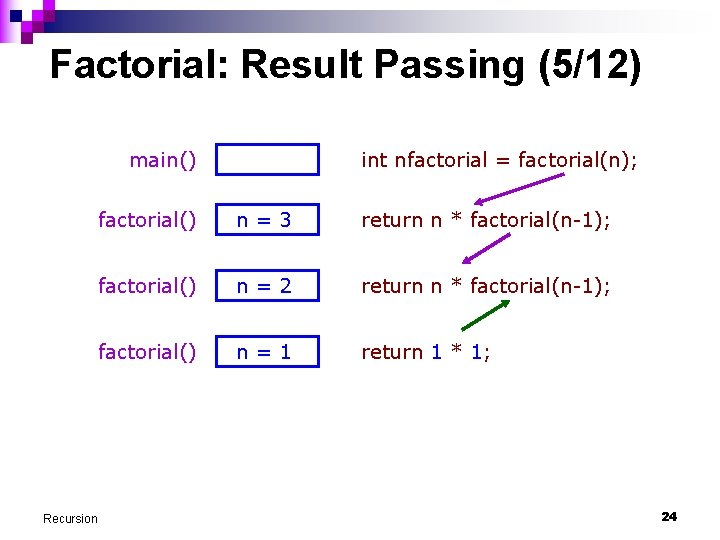Factorial: Result Passing (5/12) main() Recursion int nfactorial = factorial(n); factorial() n=3 return n * factorial(n-1); factorial() n=2 return n * factorial(n-1); factorial() n=1 return 1 * 1; 24Factorial: Result Passing (6/12) main() Recursion int nfactorial = factorial(n); factorial() n=3 return n * factorial(n-1); factorial() n=2 return n * 1; 25Factorial: Result Passing (7/12) main() Recursion int nfactorial = factorial(n); factorial() n=3 return n * factorial(n-1); factorial() n=2 return 2 * 1; 26Factorial: Result Passing (8/12) main() Recursion int nfactorial = factorial(n); factorial() n=3 return n * factorial(n-1); factorial() n=2 return 2 * 1; 27Factorial: Result Passing (9/12) main() factorial() Recursion int nfactorial = factorial(n); n=3 return n * 2; 28Factorial: Result Passing (10/12) main() factorial() Recursion int nfactorial = factorial(n); n=3 return 3 * 2; 29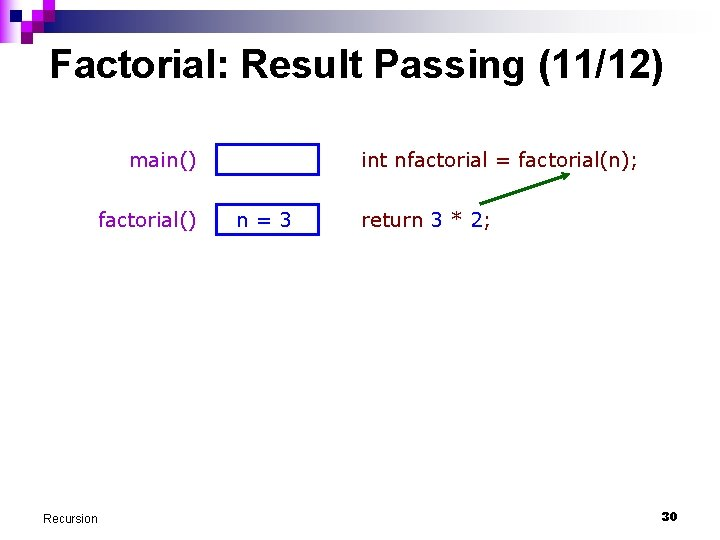Factorial: Result Passing (11/12) main() factorial() Recursion int nfactorial = factorial(n); n=3 return 3 * 2; 30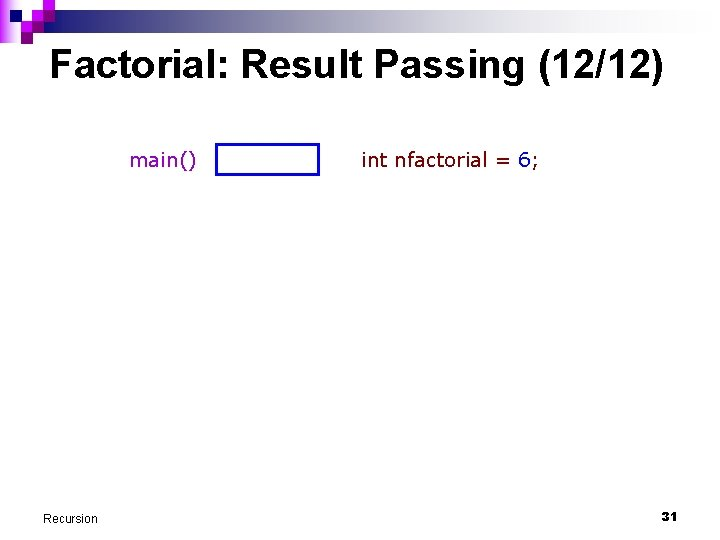Factorial: Result Passing (12/12) main() Recursion int nfactorial = 6; 31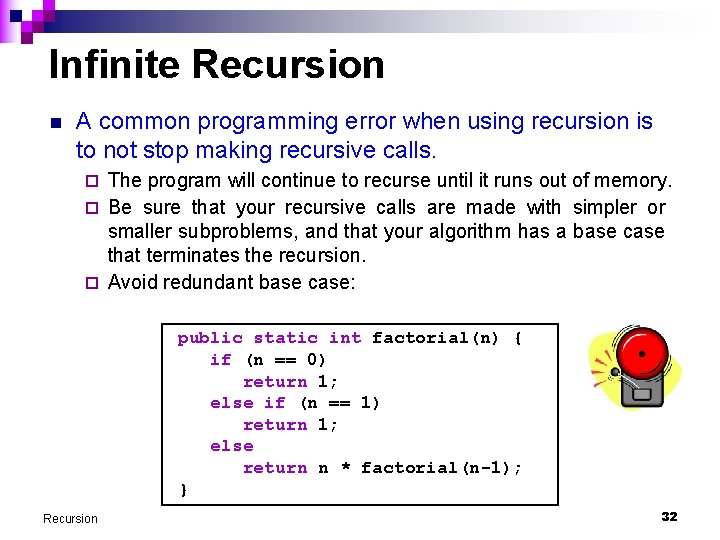Infinite Recursion n A common programming error when using recursion is to not stop making recursive calls. The program will continue to recurse until it runs out of memory. ¨ Be sure that your recursive calls are made with simpler or smaller subproblems, and that your algorithm has a base case that terminates the recursion. ¨ Avoid redundant base case: ¨ public static int factorial(n) { if (n == 0) return 1; else if (n == 1) return 1; else return n * factorial(n-1); } Recursion 32Computing Sum of Squares (1/5) n Given 2 positive integers m and n, where m ≤ n, compute: sum. Squares(m, n) = m 2 + (m+1)2 + … + n 2 n Example: sum. Squares(5, 10) = 52 + 62 + 72 + 82 + 92 + 102 = 355 Recursion 33Computing Sum of Squares (2/5) n ‘Going-up’ recursion: public static int sum. Squares (int m, int n) { if (m == n) return m * m; else return m*m + sum. Squares(m+1, n); } n ‘Going-down’ recursion: public static int sum. Squares (int m, int n) { if (m == n) return n * n; else return n*n + sum. Squares(m, n-1); } Recursion 34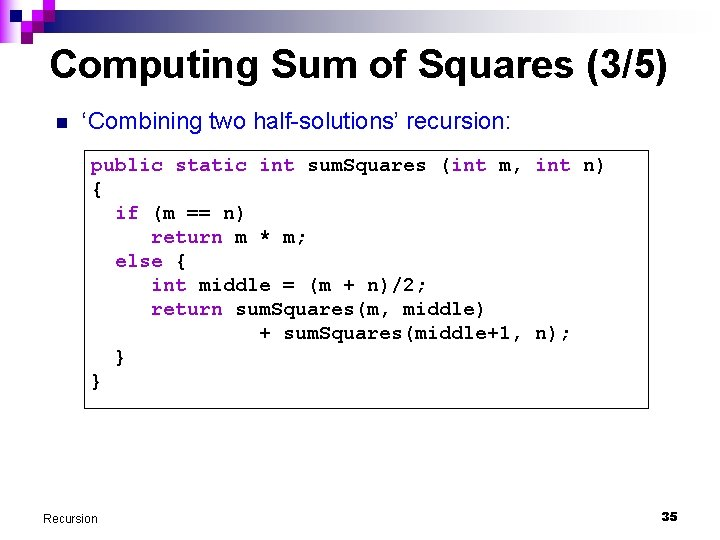Computing Sum of Squares (3/5) n ‘Combining two half-solutions’ recursion: public static int sum. Squares (int m, int n) { if (m == n) return m * m; else { int middle = (m + n)/2; return sum. Squares(m, middle) + sum. Squares(middle+1, n); } } Recursion 35Computing Sum of Squares (4/5) n Call trees for ‘going-up’ and ‘going-down’ versions. 355 sum. Squares(5, 10) 25 + 330 sum. Squares(6, 10) 36 + 294 sum. Squares(7, 10) 49 + 245 sum. Squares(8, 10) 64 + 181 sum. Squares(9, 10) 81 + 100 sum. Squares(10, 10) Recursion 100 sum. Squares(5, 10) 100 + 255 sum. Squares(5, 9) 81 + 174 sum. Squares(5, 8) 64 + 110 sum. Squares(5, 7) 49 + 61 sum. Squares(5, 6) 36 25 + sum. Squares(5, 5) 25 36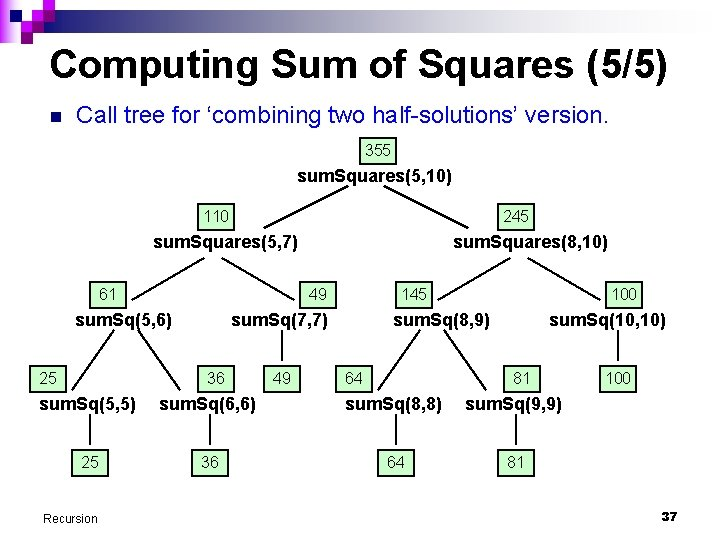Computing Sum of Squares (5/5) n Call tree for ‘combining two half-solutions’ version. 355 sum. Squares(5, 10) 110 245 sum. Squares(5, 7) 61 49 sum. Sq(5, 6) 25 sum. Squares(8, 10) 145 sum. Sq(7, 7) 36 49 100 sum. Sq(8, 9) 64 sum. Sq(10, 10) 81 sum. Sq(5, 5) sum. Sq(6, 6) sum. Sq(8, 8) sum. Sq(9, 9) 25 36 64 81 Recursion 100 37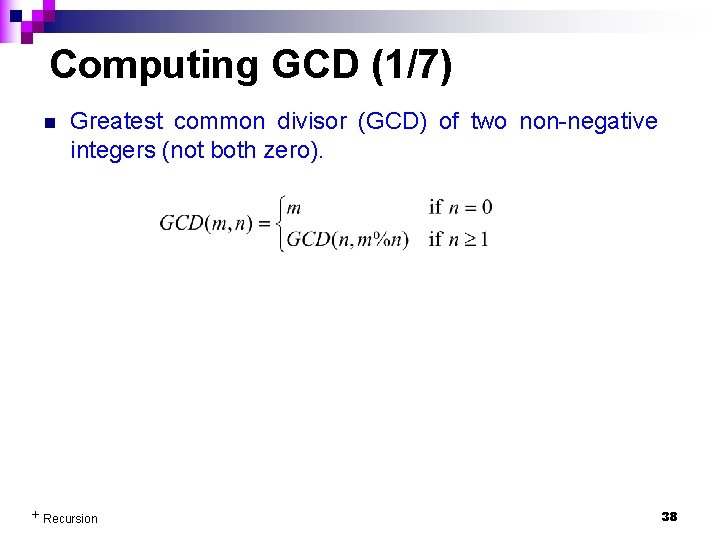Computing GCD (1/7) n Greatest common divisor (GCD) of two non-negative integers (not both zero). + Recursion 38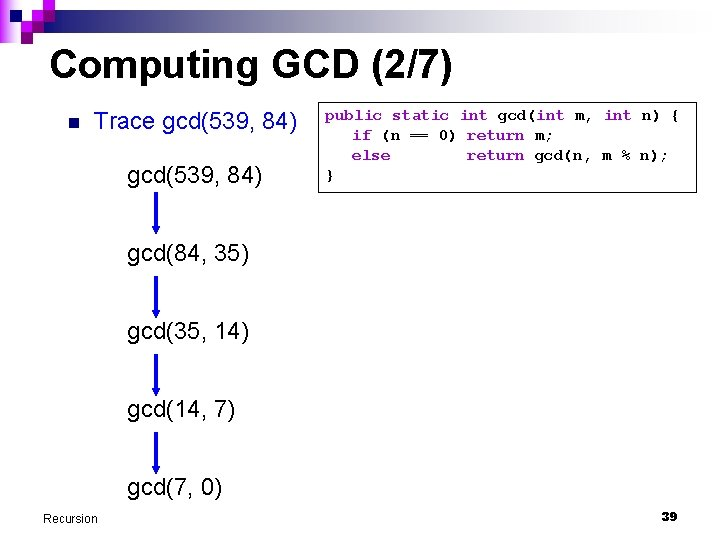Computing GCD (2/7) n Trace gcd(539, 84) public static int gcd(int m, int n) { if (n == 0) return m; else return gcd(n, m % n); } gcd(84, 35) gcd(35, 14) gcd(14, 7) gcd(7, 0) Recursion 39Computing GCD (3/7) n Trace gcd(539, 84) public static int gcd(int m, int n) { if (n == 0) return m; else return gcd(n, m % n); } gcd(84, 35) gcd(35, 14) gcd(14, 7) 7 gcd(7, 0) Recursion 40Computing GCD (4/7) n Trace gcd(539, 84) public static int gcd(int m, int n) { if (n == 0) return m; else return gcd(n, m % n); } gcd(84, 35) gcd(35, 14) 7 gcd(14, 7) Recursion 41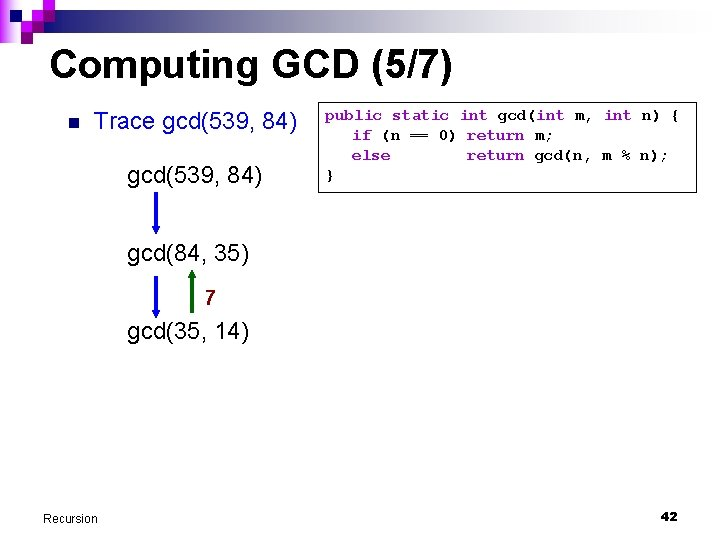Computing GCD (5/7) n Trace gcd(539, 84) public static int gcd(int m, int n) { if (n == 0) return m; else return gcd(n, m % n); } gcd(84, 35) 7 gcd(35, 14) Recursion 42Computing GCD (6/7) n Trace gcd(539, 84) public static int gcd(int m, int n) { if (n == 0) return m; else return gcd(n, m % n); } 7 gcd(84, 35) Recursion 43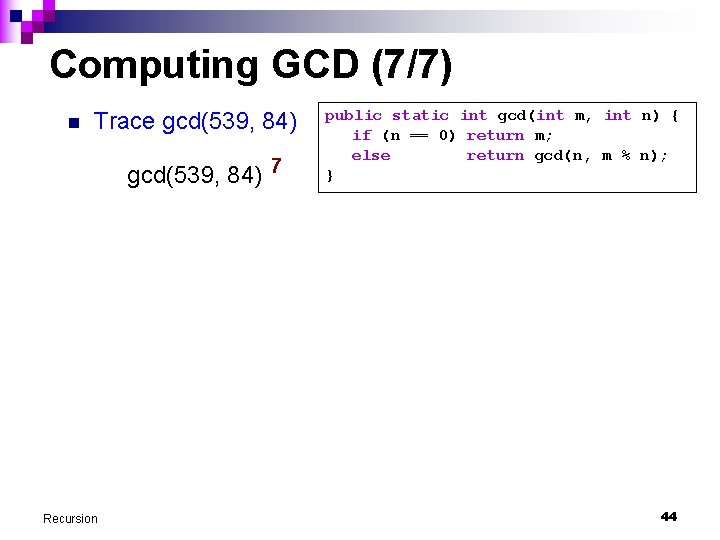Computing GCD (7/7) n Trace gcd(539, 84) 7 Recursion public static int gcd(int m, int n) { if (n == 0) return m; else return gcd(n, m % n); } 44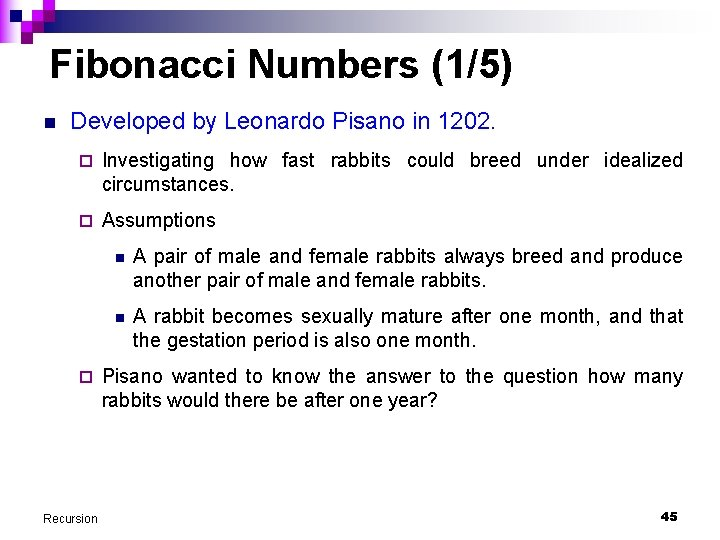Fibonacci Numbers (1/5) n Developed by Leonardo Pisano in 1202. ¨ Investigating how fast rabbits could breed under idealized circumstances. ¨ Assumptions ¨ Recursion n A pair of male and female rabbits always breed and produce another pair of male and female rabbits. n A rabbit becomes sexually mature after one month, and that the gestation period is also one month. Pisano wanted to know the answer to the question how many rabbits would there be after one year? 45Fibonacci Numbers (2/5) n The sequence generated is: 1, 1, 2, 3, 5, 8, 13, 21, 34, … n Some version starts with 0: 0, 1, 1, 2, 3, 5, 8, 13, 21, 34, … n The number of pairs for a month is the sum of the number of pairs in the two previous months. Recursion 46Fibonacci Numbers (3/5) n What is the equation for Fibonacci sequence? + Recursion 47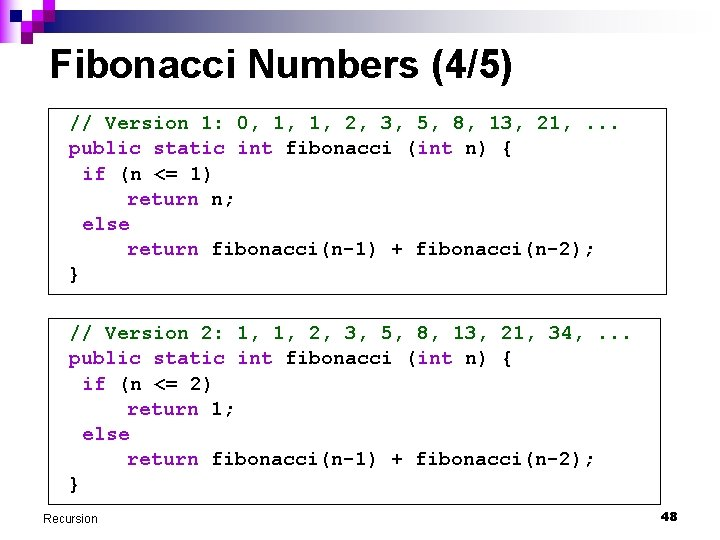Fibonacci Numbers (4/5) // Version 1: 0, 1, 1, 2, 3, 5, 8, 13, 21, . . . public static int fibonacci (int n) { if (n <= 1) return n; else return fibonacci(n-1) + fibonacci(n-2); } // Version 2: 1, 1, 2, 3, 5, 8, 13, 21, 34, . . . public static int fibonacci (int n) { if (n <= 2) return 1; else return fibonacci(n-1) + fibonacci(n-2); } Recursion 48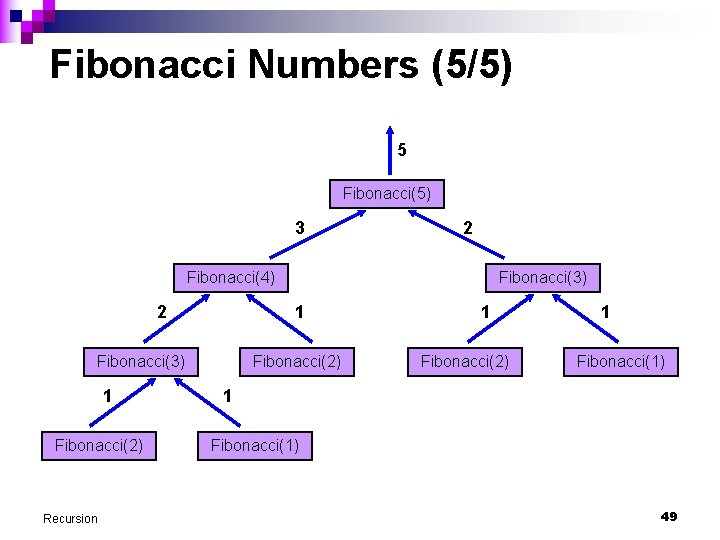Fibonacci Numbers (5/5) 5 Fibonacci(5) 3 2 Fibonacci(4) 2 1 Fibonacci(3) 1 Fibonacci(2) Recursion Fibonacci(3) Fibonacci(2) 1 Fibonacci(1) 49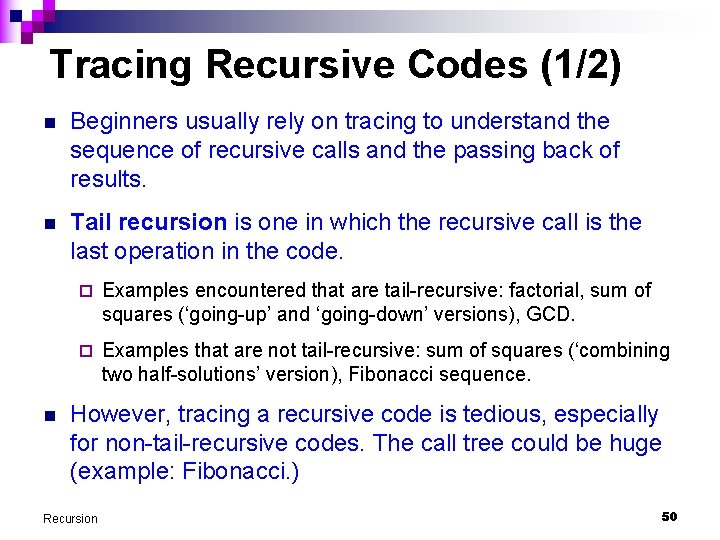Tracing Recursive Codes (1/2) n Beginners usually rely on tracing to understand the sequence of recursive calls and the passing back of results. n Tail recursion is one in which the recursive call is the last operation in the code. n ¨ Examples encountered that are tail-recursive: factorial, sum of squares (‘going-up’ and ‘going-down’ versions), GCD. ¨ Examples that are not tail-recursive: sum of squares (‘combining two half-solutions’ version), Fibonacci sequence. However, tracing a recursive code is tedious, especially for non-tail-recursive codes. The call tree could be huge (example: Fibonacci. ) Recursion 50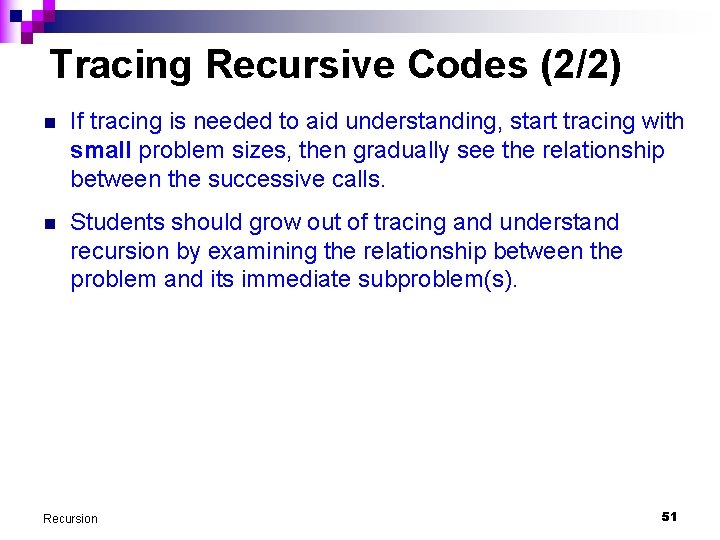Tracing Recursive Codes (2/2) n If tracing is needed to aid understanding, start tracing with small problem sizes, then gradually see the relationship between the successive calls. n Students should grow out of tracing and understand recursion by examining the relationship between the problem and its immediate subproblem(s). Recursion 51Notes n It is not typical to write a recursive main() method. n Besides direct recursion, there could be mutual or indirect recursion ¨ Examples: Method A calls method B, which calls method A. Method X calls method Y, which calls method Z, which calls method X. n Sometimes, auxiliary subroutines are needed to implement recursion. n The inherent nature of recursion gives rise to a ‘loop’ structure. At this point, most problem can be solved with such simple recursion. Recursion in a loop (or nested loops) is only needed for more advanced problems. Recursion 52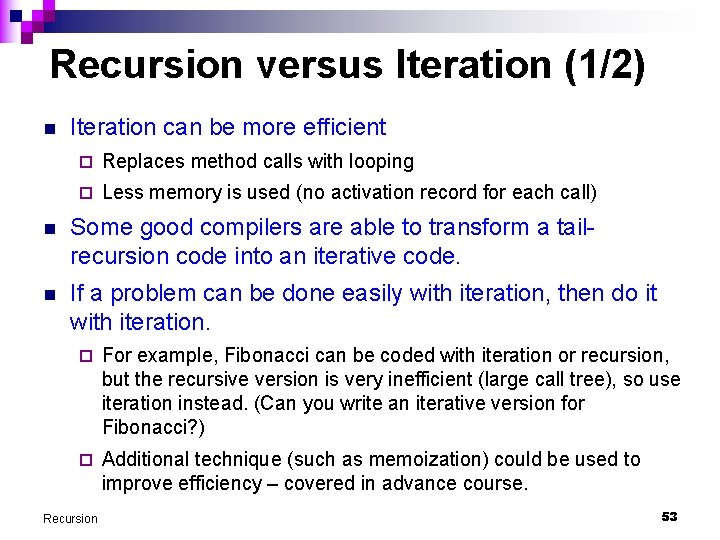Recursion versus Iteration (1/2) n Iteration can be more efficient ¨ Replaces method calls with looping ¨ Less memory is used (no activation record for each call) n Some good compilers are able to transform a tailrecursion code into an iterative code. n If a problem can be done easily with iteration, then do it with iteration. ¨ For example, Fibonacci can be coded with iteration or recursion, but the recursive version is very inefficient (large call tree), so use iteration instead. (Can you write an iterative version for Fibonacci? ) ¨ Additional technique (such as memoization) could be used to improve efficiency – covered in advance course. Recursion 53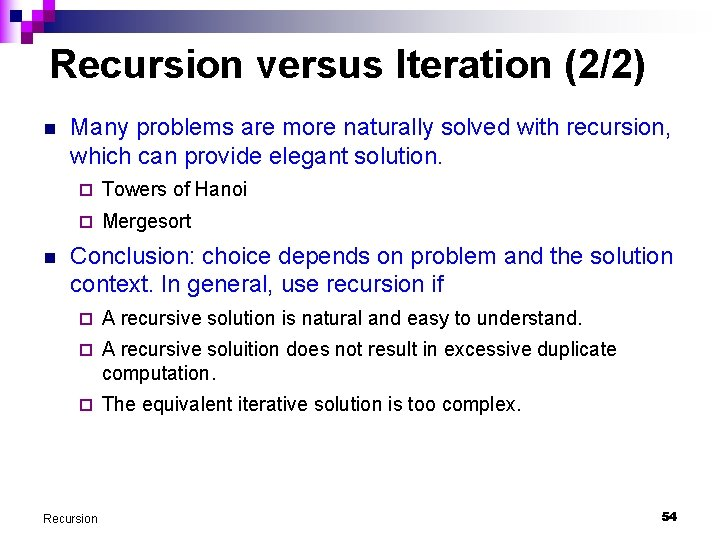Recursion versus Iteration (2/2) n n Many problems are more naturally solved with recursion, which can provide elegant solution. ¨ Towers of Hanoi ¨ Mergesort Conclusion: choice depends on problem and the solution context. In general, use recursion if ¨ A recursive solution is natural and easy to understand. ¨ A recursive soluition does not result in excessive duplicate computation. ¨ The equivalent iterative solution is too complex. Recursion 54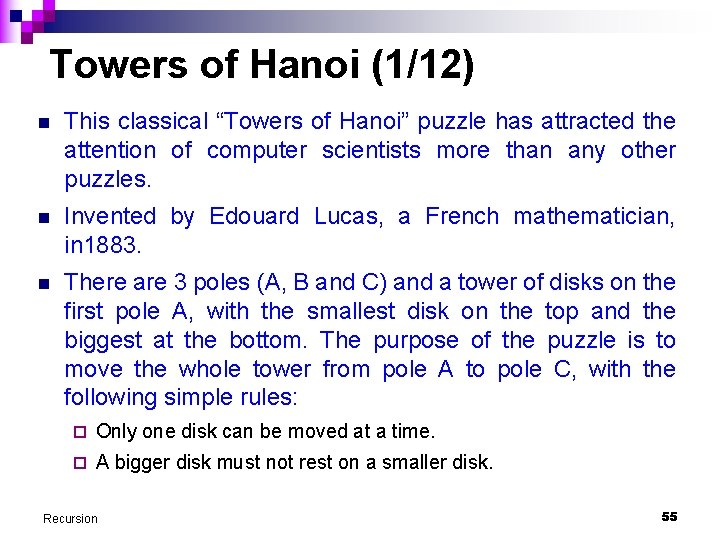Towers of Hanoi (1/12) n This classical “Towers of Hanoi” puzzle has attracted the attention of computer scientists more than any other puzzles. n Invented by Edouard Lucas, a French mathematician, in 1883. n There are 3 poles (A, B and C) and a tower of disks on the first pole A, with the smallest disk on the top and the biggest at the bottom. The purpose of the puzzle is to move the whole tower from pole A to pole C, with the following simple rules: ¨ Only one disk can be moved at a time. ¨ A bigger disk must not rest on a smaller disk. Recursion 55Towers of Hanoi (2/12) n n Legend: ¨ In the great temple of Brahma in Benares, on a brass plate under the dome that marks the center of the world, there are 64 disks of pure gold that the priests carry one at a time between these diamond needles according to Brahma's immutable law: No disk may be placed on a smaller disk. In the begging of the world all 64 disks formed the Tower of Brahma on one needle. Now, however, the process of transfer of the tower from one needle to another is in mid course. When the last disk is finally in place, once again forming the Tower of Brahma but on a different needle, then will come the end of the world and all will turn to dust. ¨ Reference: R. Douglas Hofstadter. Metamagical themas. Scientific American, 248(2): 16 -22, March 1983. Demo: Tower of Hanoi Recursion 56Towers of Hanoi (3/12) n We attempt to write a produce to produce instructions on how to move the disks from pole A to pole C to complete the puzzle. n Example: A tower with 3 disks. n Output produced by program is as followed. It is assumed that only the top disk can be moved. Move disk from A to C Move disk from A to B Move disk from C to B Move disk from A to C Move disk from B to A Move disk from B to C Move disk from A to C Recursion 57Towers of Hanoi (4/12) n Example: A tower with 3 disks. Move disk from A to C Move disk from A to B Move disk from C to B Move disk from A to C Move disk from B to A Move disk from B to C Move disk from A to C A Recursion B C 58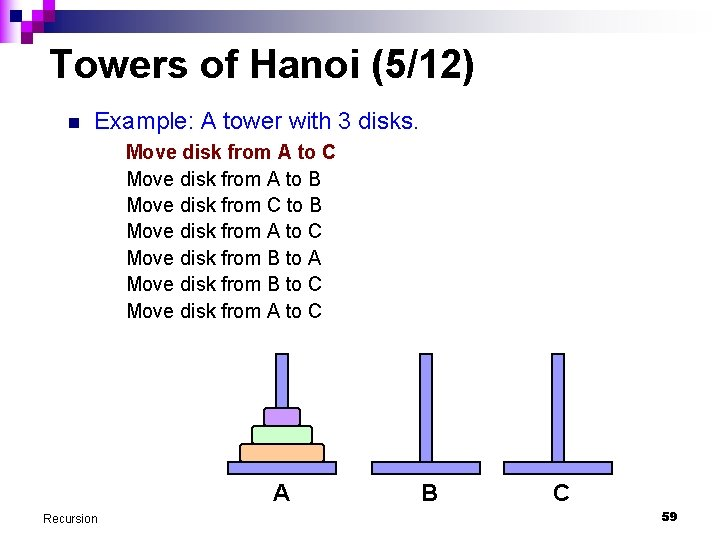Towers of Hanoi (5/12) n Example: A tower with 3 disks. Move disk from A to C Move disk from A to B Move disk from C to B Move disk from A to C Move disk from B to A Move disk from B to C Move disk from A to C A Recursion B C 59Towers of Hanoi (6/12) n Example: A tower with 3 disks. Move disk from A to C Move disk from A to B Move disk from C to B Move disk from A to C Move disk from B to A Move disk from B to C Move disk from A to C A Recursion B C 60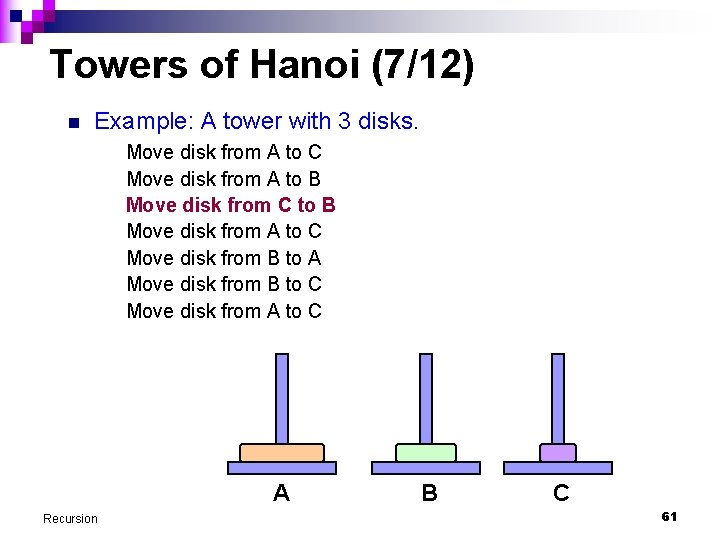Towers of Hanoi (7/12) n Example: A tower with 3 disks. Move disk from A to C Move disk from A to B Move disk from C to B Move disk from A to C Move disk from B to A Move disk from B to C Move disk from A to C A Recursion B C 61Towers of Hanoi (8/12) n Example: A tower with 3 disks. Move disk from A to C Move disk from A to B Move disk from C to B Move disk from A to C Move disk from B to A Move disk from B to C Move disk from A to C A Recursion B C 62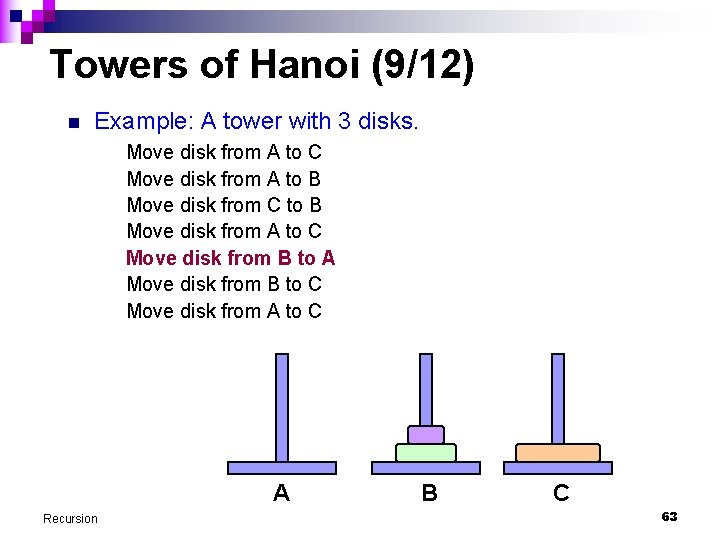Towers of Hanoi (9/12) n Example: A tower with 3 disks. Move disk from A to C Move disk from A to B Move disk from C to B Move disk from A to C Move disk from B to A Move disk from B to C Move disk from A to C A Recursion B C 63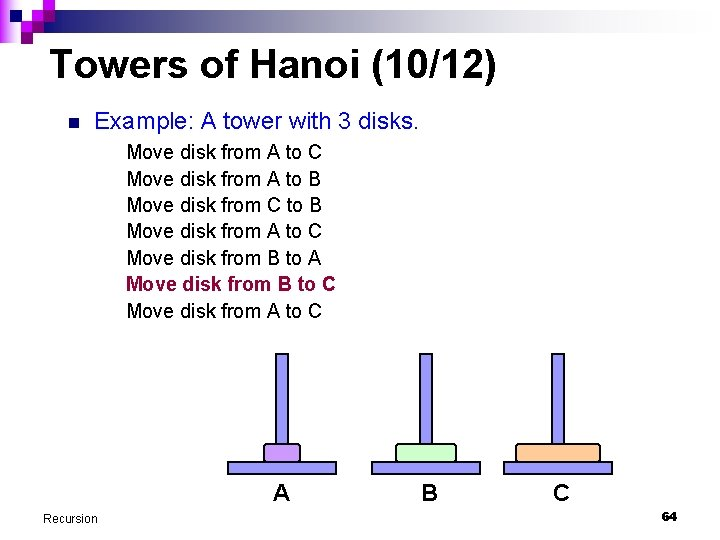Towers of Hanoi (10/12) n Example: A tower with 3 disks. Move disk from A to C Move disk from A to B Move disk from C to B Move disk from A to C Move disk from B to A Move disk from B to C Move disk from A to C A Recursion B C 64Towers of Hanoi (11/12) n Example: A tower with 3 disks. Move disk from A to C Move disk from A to B Move disk from C to B Move disk from A to C Move disk from B to A Move disk from B to C Move disk from A to C A Recursion VIOLA! B C 65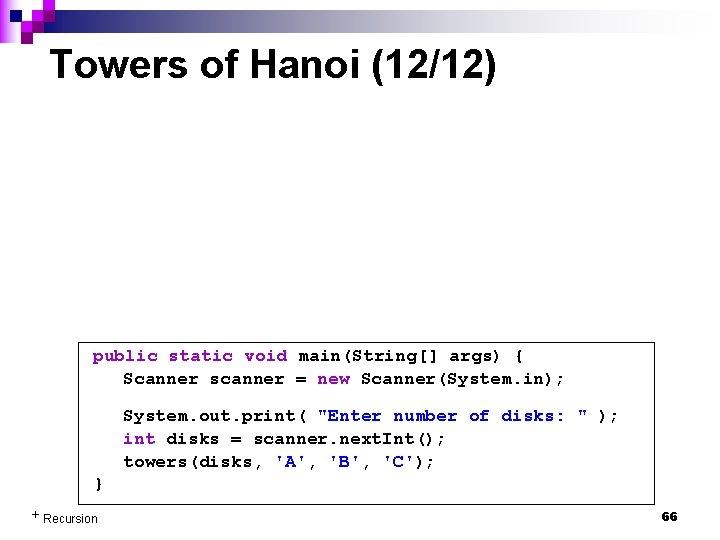Towers of Hanoi (12/12) public static void main(String[] args) { Scanner scanner = new Scanner(System. in); System. out. print( "Enter number of disks: " ); int disks = scanner. next. Int(); towers(disks, 'A', 'B', 'C'); } + Recursion 66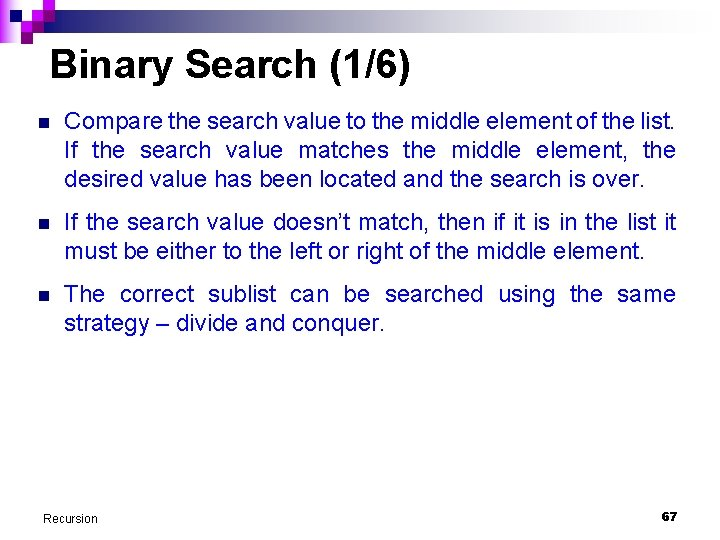Binary Search (1/6) n Compare the search value to the middle element of the list. If the search value matches the middle element, the desired value has been located and the search is over. n If the search value doesn’t match, then if it is in the list it must be either to the left or right of the middle element. n The correct sublist can be searched using the same strategy – divide and conquer. Recursion 67Binary Search (2/6) data           3 6 8 12 17 20 21 32 33 45 Compare search value to this element. If the search value matches, the search is successful. If the search value is less than this element (data), then the search value, if it is in the list, must be to the left of data. If the search value is greater than this element (data), then if it is in the list, it must be to the right of data. Recursion 68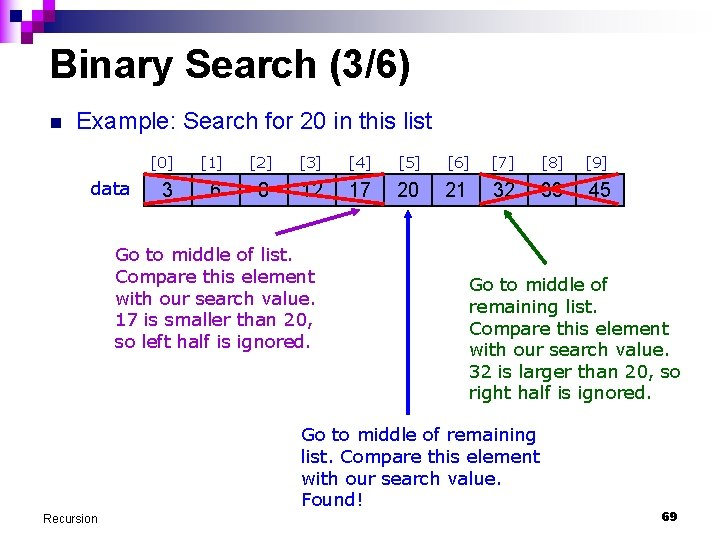Binary Search (3/6) n Example: Search for 20 in this list data           3 6 8 12 17 20 21 32 33 45 Go to middle of list. Compare this element with our search value. 17 is smaller than 20, so left half is ignored. Go to middle of remaining list. Compare this element with our search value. 32 is larger than 20, so right half is ignored. Go to middle of remaining list. Compare this element with our search value. Found! Recursion 69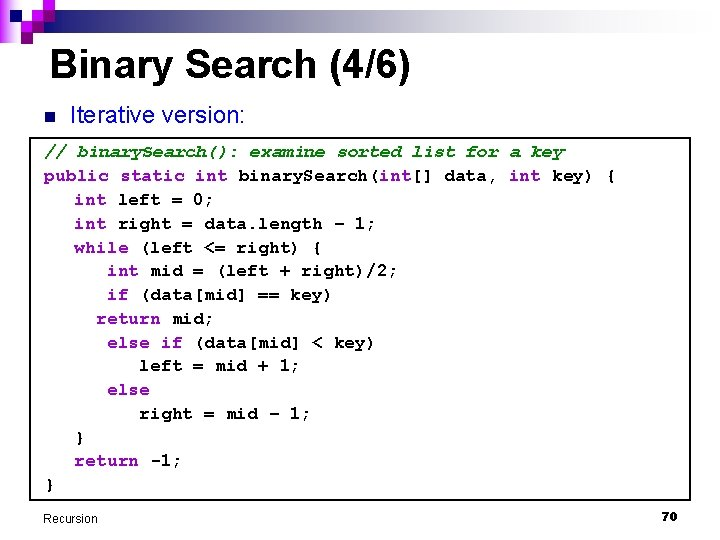Binary Search (4/6) n Iterative version: // binary. Search(): examine sorted list for a key public static int binary. Search(int[] data, int key) { int left = 0; int right = data. length – 1; while (left <= right) { int mid = (left + right)/2; if (data[mid] == key) return mid; else if (data[mid] < key) left = mid + 1; else right = mid – 1; } return -1; } Recursion 70Binary Search (5/6) n Recursive version: + Recursion 71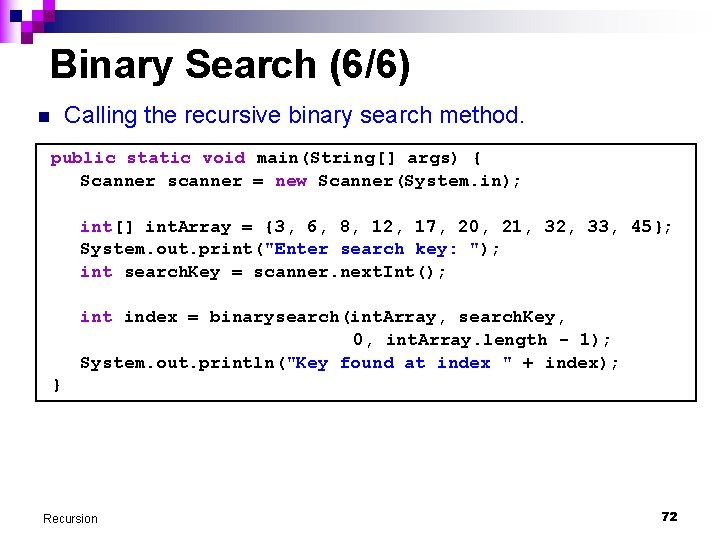Binary Search (6/6) n Calling the recursive binary search method. public static void main(String[] args) { Scanner scanner = new Scanner(System. in); int[] int. Array = {3, 6, 8, 12, 17, 20, 21, 32, 33, 45}; System. out. print("Enter search key: "); int search. Key = scanner. next. Int(); int index = binarysearch(int. Array, search. Key, 0, int. Array. length - 1); System. out. println("Key found at index " + index); } Recursion 72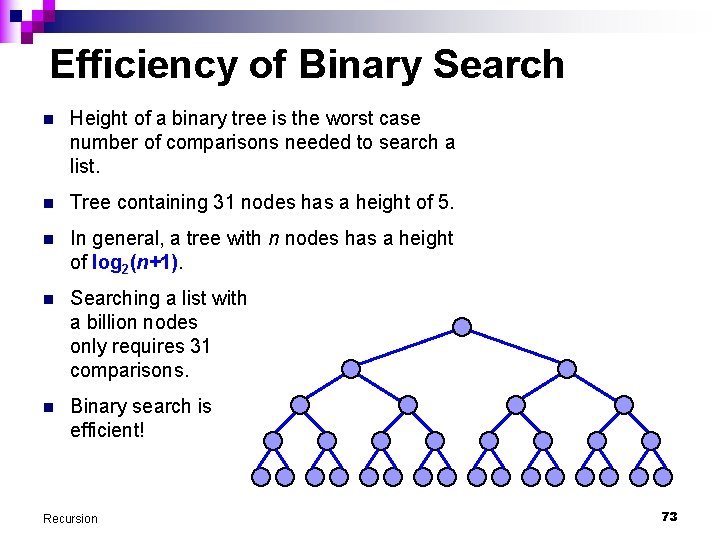Efficiency of Binary Search n Height of a binary tree is the worst case number of comparisons needed to search a list. n Tree containing 31 nodes has a height of 5. n In general, a tree with n nodes has a height of log 2(n+1). n Searching a list with a billion nodes only requires 31 comparisons. n Binary search is efficient! Recursion 73Recursion on Data Structures n Besides using recursion to compute numerical values (factorial, Fibonacci numbers, etc. ), recursion is also useful for processing on data structures (example: arrays). n Examples: n ¨ Binary search on a sorted array. ¨ Finding maximum or minimum value in an array. ¨ Finding a path through the maze. ¨ And many others… Some of the more complex problems belong to a more advanced course, but we shall look at some simpler problems. Recursion 74Reversing an Array // reverse an integer array public static void reverse (int[] array, int start, int finish) { if (start < finish) { int temp = array[start]; array[start] = array[finish]; array[finish] = temp; reverse (array, start+1, finish-1); } } public static void main(String[] args) { int[] int. Array = { 3, 7, 1, 4, 12, 9, 7, 5 }; reverse (int. Array, 0, int. Array. length-1); } Recursion 75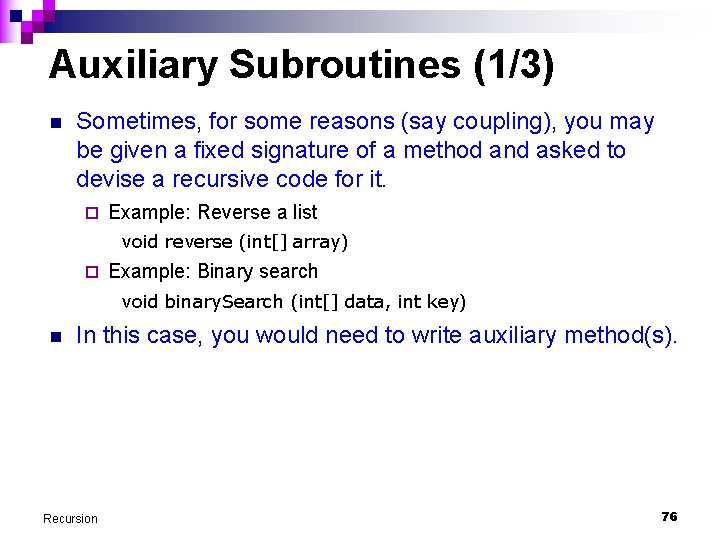Auxiliary Subroutines (1/3) n Sometimes, for some reasons (say coupling), you may be given a fixed signature of a method and asked to devise a recursive code for it. ¨ Example: Reverse a list void reverse (int[] array) ¨ Example: Binary search void binary. Search (int[] data, int key) n In this case, you would need to write auxiliary method(s). Recursion 76Auxiliary Subroutines (2/3) n Reversing a list // reverse an integer array public static void reverse (int[] array) { reverse. Aux (array, 0, array. length-1); } // reverse an integer array private static void reverse. Aux (int[] array, int start, int finish) { if (start < finish) { int temp = array[start]; array[start] = array[finish]; array[finish] = temp; reverse. Aux (array, start+1, finish-1); } } Recursion 77Auxiliary Subroutines (3/3) n The call to reverse() method is then simplified as follows. ¨ Note that this does not require the caller to provide the starting and ending indices as in the previous version. This reduces coupling. public static void main(String[] args) { int[] int. Array = { 3, 7, 1, 4, 12, 9, 7, 5 }; reverse (int. Array); } ¨ Note that the auxiliary method reverse. Aux() is declared as private, since it is only to be called by the reverse() method and not by any other methods. ¨ Note also that the auxiliary method could also be named reverse(), since Java allows method overloading. Recursion 78Optional n The following slides are for your reading. n There are sorting algorithms that employ recursion: quicksort, mergesort. These are faster sorting algorithms which will be covered in another module (CS 1102). n Previous examples use simple recursion. The recursive call substitute the loop in the iterative counterpart. n The following examples (directory listing, anagrams) are slightly more complex, requiring the combination of a loop and recursive call. Recursion 79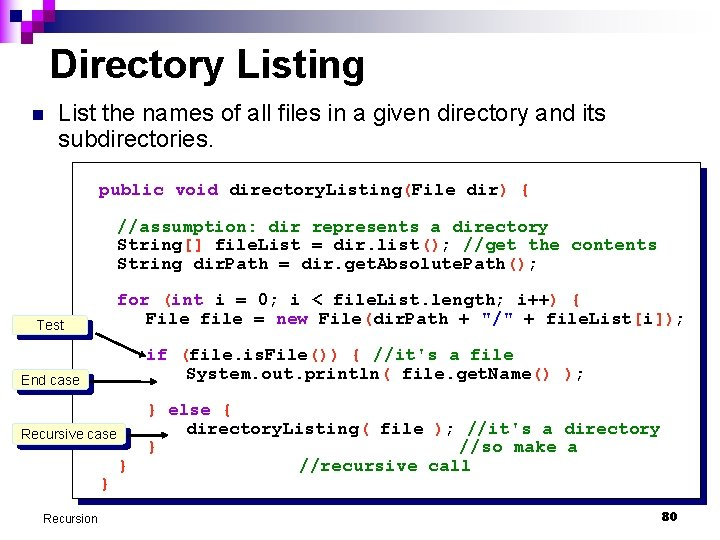Directory Listing n List the names of all files in a given directory and its subdirectories. public void directory. Listing(File dir) { //assumption: dir represents a directory String[] file. List = dir. list(); //get the contents String dir. Path = dir. get. Absolute. Path(); for (int i = 0; i < file. List. length; i++) { File file = new File(dir. Path + "/" + file. List[i]); Test if (file. is. File()) { //it's a file System. out. println( file. get. Name() ); End case Recursive case } Recursion } } else { directory. Listing( file ); //it's a directory } //so make a //recursive call 80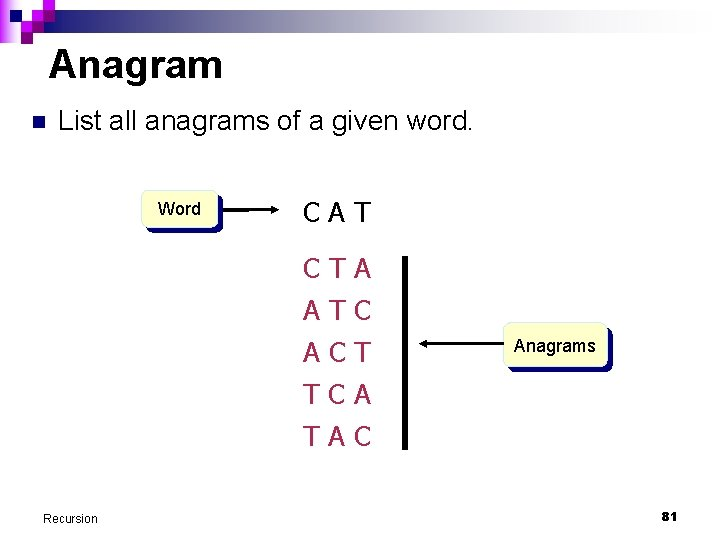Anagram n List all anagrams of a given word. Word CAT CTA ATC ACT Anagrams TCA TAC Recursion 81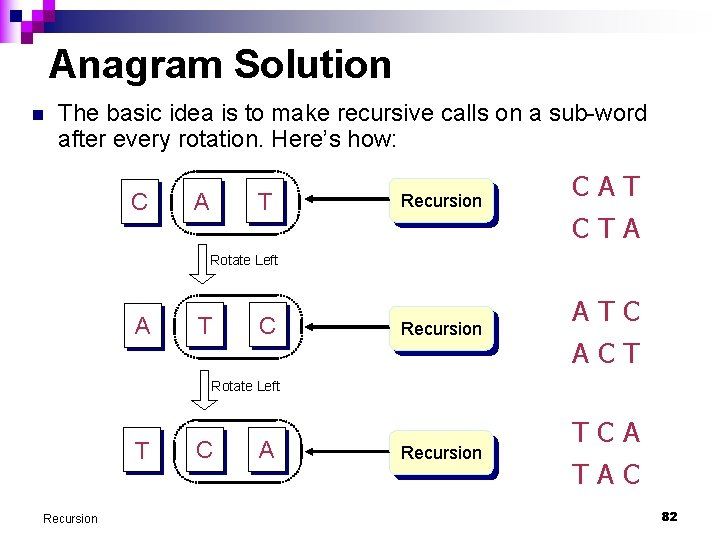Anagram Solution n The basic idea is to make recursive calls on a sub-word after every rotation. Here’s how: C A T Recursion CAT CTA Recursion ATC ACT Recursion TCA TAC Rotate Left A T C Rotate Left T Recursion C A 82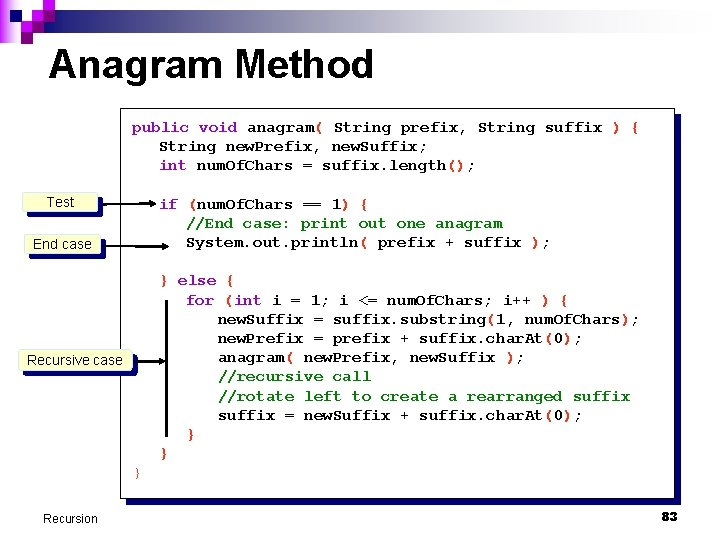Anagram Method public void anagram( String prefix, String suffix ) { String new. Prefix, new. Suffix; int num. Of. Chars = suffix. length(); Test if (num. Of. Chars == 1) { //End case: print out one anagram System. out. println( prefix + suffix ); End case } else { for (int i = 1; i <= num. Of. Chars; i++ ) { new. Suffix = suffix. substring(1, num. Of. Chars); new. Prefix = prefix + suffix. char. At(0); anagram( new. Prefix, new. Suffix ); //recursive call //rotate left to create a rearranged suffix = new. Suffix + suffix. char. At(0); } } Recursive case } Recursion 83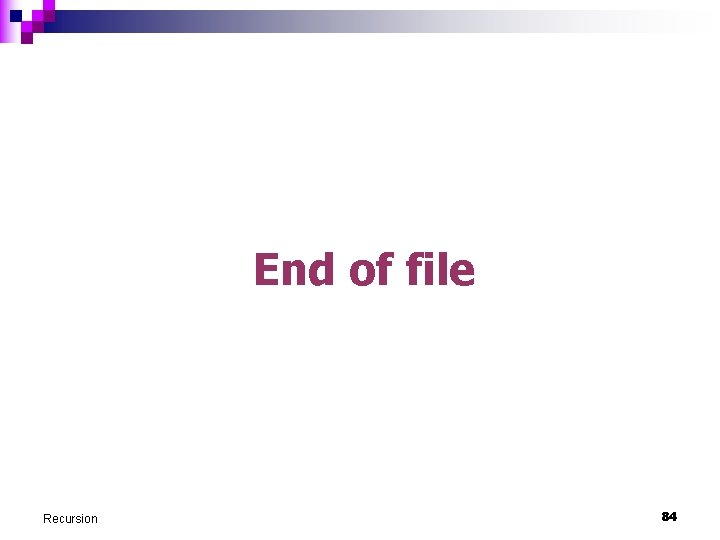End of file Recursion 84